Select Page
##### Scatter diagram – 7QC Tools

Scatter diagram is a tool for analyzing relationships between two variables. In the two variables chosen one variable is plotted on the horizontal axis and the other variable is plotted on the vertical axis. The pattern of their intersecting points can graphically show the relationship patterns between the two variables.

Mostly the scatter diagram is used to prove or disprove cause-and-effect relationships between two variables which helps in decision making during the Problem Solving process.

To understand whether there is any relationship between the atmospheric temperature and the Ice cream sales figure, daily atmospheric temperature can be considered as one variable and the daily sales revenue can be considered as the second variable. These data can be plotted using a scatter diagram and the relationships can be inferred from it.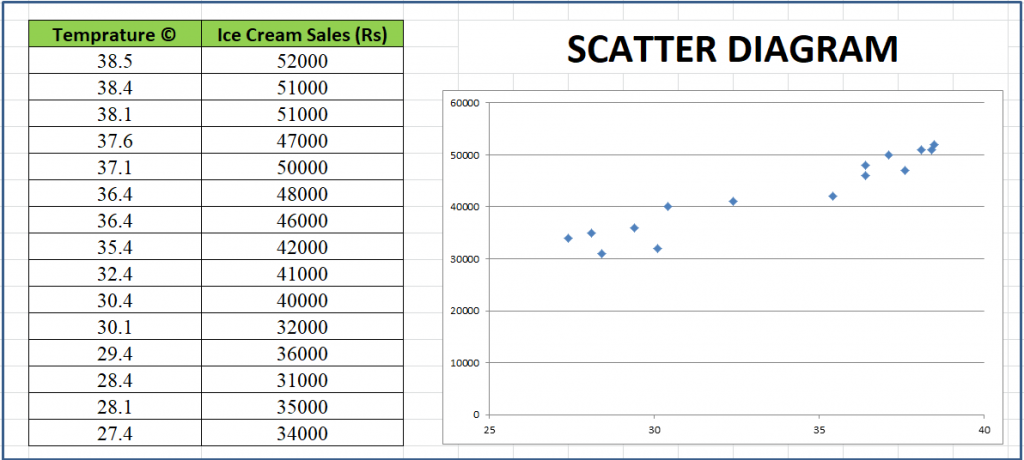The above example indicates that there is a reasonable increase in the ice cream sales with respect to the daily temperature. The scatter Diagram indicates that the the trend of the sales is on increase with respect to temperature. This diagram proves that the there exist a relationship between the daily temperature and the ice cream sales which is also a positive correlation.

The relationship varies by the nature of the correlation. The various type of correlation that can possibly exist are detailed below.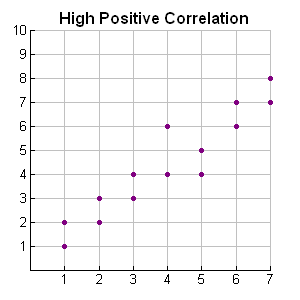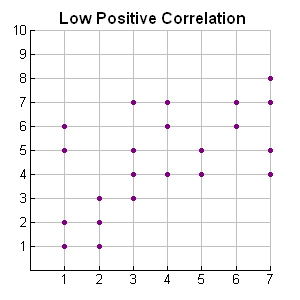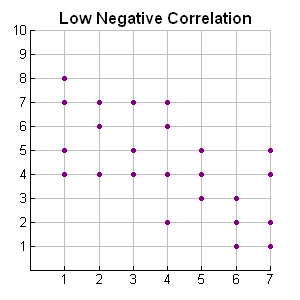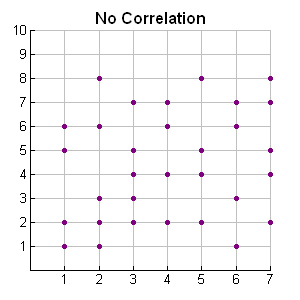When two variables are put into scatter diagram the obvious results fall into any of the above five correlations. These information helps us to take decisions during the problem solving process.

When we consider a machine shop, if there is a doubt that the coolant concentration affects the surface finish and needs some clarity if the relationship does really exists to take further decisions a Scatter Diagram can be used as a tool to prove or disprove the existence of the relationship. Here Coolant concentration and Roughness average becomes two variables and correlations can be verified.

Even when this is considered a important tool in arriving at decisions we must be very careful in choosing the variables to compare. If we take two variables such as TV Sales figure Vs Hand Bag sales Figure and want to know if there is any relationship between these two it is obviously going to be of no use from the correlation. Because the two variables does not infer with each other logically. Instead if there is a comparison to between the two variables such as TV Sales Figure Vs Stabilizer sales Figure the two variables has some logic to do so. Therefore it is important to carefully select the variables to have an effective results from the Scatter Diagram.

We believe the content posted above has helped you to learn the basic explanation about the Scatter Diagram which is one of the 7 QC tools used for problem solving. We would be glad to hear your comments in the comments section below.

Few books recommended to read on 7QC Tools are given below